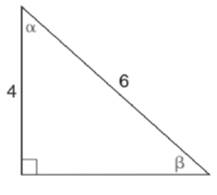Chapter 11.3, Problem 3EElementary Geometry For College St...

7th Edition
Alexander + 2 others
ISBN: 9781337614085

Solutions

Chapter
SectionElementary Geometry For College St...

7th Edition
Alexander + 2 others
ISBN: 9781337614085
Textbook Problem

In Exercises 1 to 4, find tan α and tan β for each triangle.To determine

To find:

The values of tanα and tanβ for the triangle.

Explanation

Given:

The triangle is shown below.

Definition used:

In a right triangle, the tangent ratio for an acute angle is the ratio oppositeadjacent.

Calculation:

In a right triangle, (hypotenuse)2=(opposite)2+(adjacent)2

Let x be the unknown side in the given figure.

(6)2=x2+4236=x2+163616=x220=x220=x25=x

Therefore, the unknown side is x=25

Still sussing out bartleby?

Check out a sample textbook solution.

See a sample solution

The Solution to Your Study Problems

Bartleby provides explanations to thousands of textbook problems written by our experts, many with advanced degrees!

Get Started

Simplify: 330360

Elementary Technical Mathematics

Find the mean, median, and mode for the following scores; 8, 7, 5, 7, 0, 10, 2, 4, 11, 7, 8, 7

Essentials of Statistics for The Behavioral Sciences (MindTap Course List)

In Exercises 31-34, find an equation of the line that passes through the points. 34. (1, 2) and (3, 4)

Applied Calculus for the Managerial, Life, and Social Sciences: A Brief Approach

Describe the scores in a sample that has standard deviation of zero.

Statistics for The Behavioral Sciences (MindTap Course List)

Simplify the expressions in Exercises 97106. (xy)1/3(yx)2/3

Finite Mathematics and Applied Calculus (MindTap Course List)

True or False: f(x)f(a)xa may be interpreted as the instantaneous velocity of a particle at time a.

Study Guide for Stewart's Single Variable Calculus: Early Transcendentals, 8th